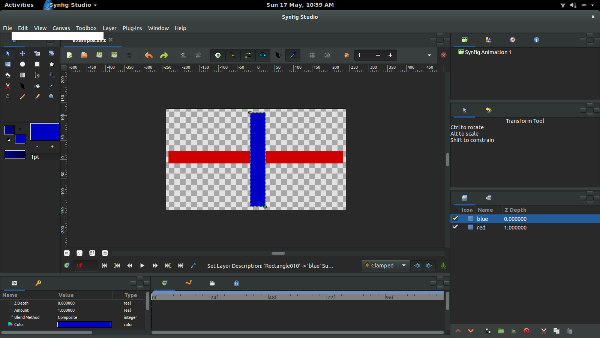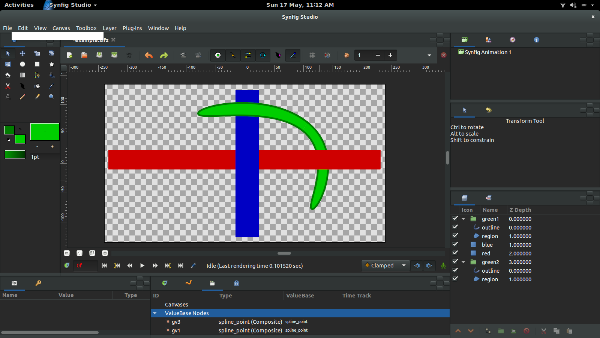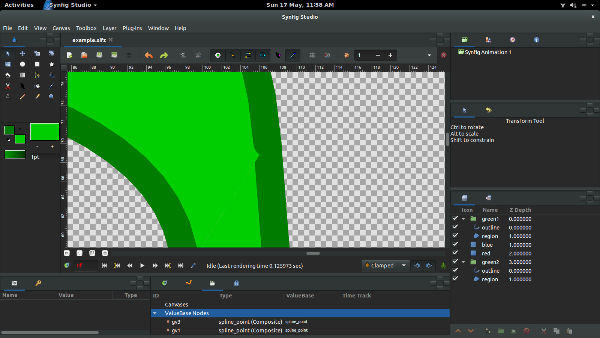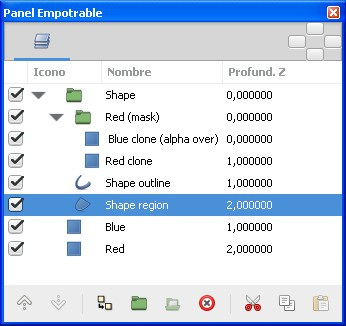# How to make an object spanning multiple layers

I need to make an object that goes in front of the blue rectangle and behind the red rectangle in something like the following picture.My attempt at doing this is as follows:

1. Create two green splines. One will be above the blue rectangle, and the other will be below the red rectangle.
2. Combine the splines by exporting the two open vertices on one spline and converting the two vertices on the other spline to reverse tangent.
3. Connect the corresponding exported vertices with the reference sub-parameters and check reverse.

Which produces the following:This seems fine at first, however I have the following problems with it:

1. I can only use the vertex of the first spline when editing the tangent. Also I can’t split the tangent’s radius, which I would like to be able to do.
2. There is a noticeable gap between the regions of the two splines.
3. Depending on how the tangent’s are positioned, the region of the upper spline will overlap the outline of the lower spline:example.sifz (2.05 KB)

The gap is not possible to avoid with your setup. It will appear in one or other position depending on the relative position of the points of the shape cut.

You can do it opposite. Instead of split the shape do this composition:example3.sifz (2.31 KB)
-G